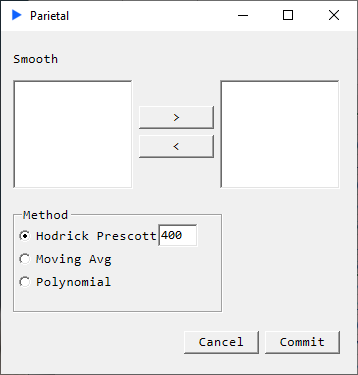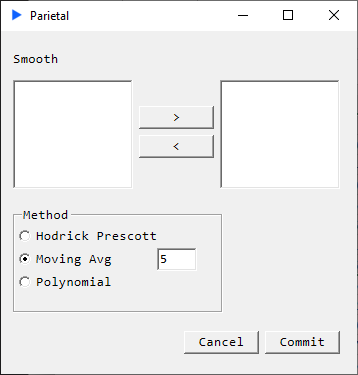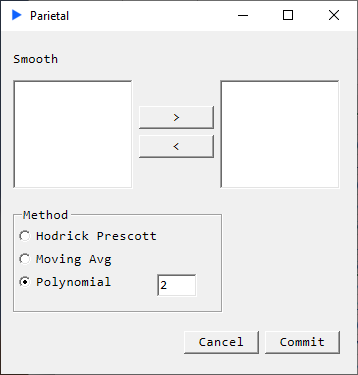# Smoothing

## Method: Hodrick Prescott Filter###### Description#

Return a matrix that has been smoothed column-wise using the Hodrick Prescott filter. In other words, the HP filter extracts the trend component from a time series. Our implementation of the filter here makes two passes, forward and backward.

$$\large min_{\tau}(\sum_{t=1}^T\left(y_t - \tau_t\right)^2 + \lambda \sum_{t=2}^{T-1}\left((\tau_{t+1} - \tau_t) - (\tau_t - \tau_{t-1})\right)^2)$$

 voxeu.org

 bis.org

##### Returns#
• Smoothed matrix. I.e. a matrix with trend components, cyclical components are ignored.

## Method: Moving Average###### Description#

Return a matrix that has been smoothed column-wise using a simple moving average.

##### Returns#
• Smoothed matrix.

## Method: Polynomial###### Description#

Return a matrix that has been smoothed column-wise. The method fits a polynomial of a user specified order, decomposes the vector into trend and cycle components but only returns the trend component.

##### Returns#
• Smoothed matrix.